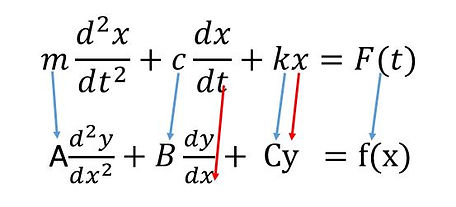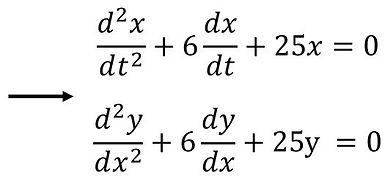naver-site-verification: naver47238a1b6bfbb19a2fd4b619734fa9a6.html
top of page

# Analysis of a Mass spring system with damping

## Analyzed with a second order differential equation solver

Mass springs systems are common components of mechanical and electrical systems as a part of the whole system. Therefore, it is important for electrical and mechatronic engineers to gain insight into their behavior. On this page, the dynamic behavior of a mass spring system is discussed.

The study of the frequency response of a mass spring system is discussed on a special page, namely:

On this page, we start with a one-dimensional mass spring system with damping. With the second-order differential equation solver program, the dynamic behavior for different values of stiffness, damping and mass can be easily analyzed.For the system, the dynamic force equation is shown.

The second order differential equations can be solved analytically, but to gain insight quickly into the influence of various parameters the program DV2ETRAP.8xp can be a great help.

As a first example, we take m=1 kg for the mass, c=6 Nm/s for the damping and k=25 N/m for the stiffness. The force F(t)=0 N but the initial condition for t=0 s : x(0)=4 m. The differential equation solver of the Ti84

solves.Therefore, A=m=1, B=C=6, C=k=25  and f(x) =F(t) and represents x, x represents t## Analytical solution

As F(t), f(x)=0 only a homogenous differential equation with an initial condition is left (x(0)=4 m) . it can be shown that for the analytical solution holds : x(t) = 4e^(-3t)cos(4t)+3e^(-3t)sin(4t) m

Before solving the differential equation with the calculator, we plot the analytical solution with the Ti84 plus in Y1. The mass is is strongly damped and comes to a standstill very quickly within 1.5 sec.## Application of the Ti-84 plus second order differential solver

On the page second order differential equation solver, you can find extensive information about the program.

An important parameter that the program asks for is the step size. For a second order system, the resonance frequency for a system without damping = √(k/m)=√(25/1)=5 rad/sec is about 1 Hz.

To sample a signal with a frequency of 1 Hz, we have to sample 1 period at least 20 times, so dx< 1/20. We take dx=1/50. For a maximum time of 5 sec. the calculator has to do 5*50=250 runs which is an easy job for your Ti. (Avoid getting more than 2000 runs). The input of the program and the plots calculated analytically and by the Ti are shown.The plots show that the numerical solver gives very good results. For the point x=1.02 the difference between analytical and numerical result is 0.224318-0.22511=0.000792.

Now let us decrease the damping with a factor of 20 to c=0.3Nm/s. We have seen that we can trust the results of the numerical solver, so no analytical result is derived.### Response of a mass spring system with very low damping

For a small damping, the amplitude hardly decreases as function of time. According to the plots, the period time is 2.52-1.26=1.26 sec. Resonance frequency is calculated at √25=5 rad/sec =5/(2π)= 0.7957Hz which is a period time of 1.2566 sec (rounded 1.26 sec). The result turns out to be correct.

Finally, an example with step function F(t) = 100 N. Mass m= 1 kg, damping 6Nm/s and stiffness again 25 N/m initial conditions x(0) and dx(0)/dt equals 0. The mass position has some overshoot (about 10 %) and ends at a value of 4 m. This value equals F/k=100/25=4 m which is the steady state situation (no speed and acceleration).### Step response of a mass spring system with subcritical damping and overshoot

To avoid overshoot, the system should have critical damping. This holds for c^2-4km=0, c^2=4km=100 therefore c=10Nm/s. Further, increasing of damping (15Nm/s) results in a slow system. The driving force is still 100 N.### Step response of a mass spring system with critical and supercritical damping

If you are  interested in the second order differential solver for your Ti, then send a mail to frieboon@gmail.com with your request.

If you are interested in the Bode diagram analysis, than go to:

measured since 8-11-2022

bottom of page